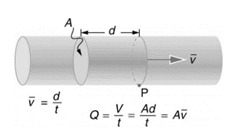Chapter 12, Problem 1CQ

Chapter
Section
Textbook Problem

What is the difference between flow rate and fluid velocity? How are they related?

To determine

The difference between flow rate and fluid velocity along with its relation

Flow rate is given by

Q=Vt

Fluid velocity is given by

v=dt

Explanation

Introduction: Flow rate

can also be defined as volume flow rate, it is a scalar quantity.

Fluid velocity

is a vector quantity.Flow rate

Q is defined as volume of fluid V passes through cross section A per unit time t.

Mathematically it is given by equation,

Q=Vt

SI unit -

m3s

CGS unit -

cm3s

Fluid velocity

vis defined as distance travelled by fluid d in certain interval of time t.

Mathematically it is given by equation,

v=dt

SI unit -

ms

CGS unit -

cms

Relation between Flow rate & Fluid velocity Flow rate can be given as

Q=Vt

Flow rate = Area of cross section x Fluid velocity.

Conclusion:

The greater the velocity of flow, greater the flow rate.

Flow rate is given by

Q=Vt

Fluid velocity is given by

v=dt

The Solution to Your Study Problems

Bartleby provides explanations to thousands of textbook problems written by our experts, many with advanced degrees!

Get Started

What is motion?

An Introduction to Physical Science

List the differences between mitosis and meiosis in the following chart:

Human Heredity: Principles and Issues (MindTap Course List)

Find all the answers to your study problems with bartleby.
Textbook solutions plus Q&A. Get As ASAP arrow_forward